Coefficient, CoefficientList

In the next cell Coefficient returns the coefficient of a particular term of a polynomial.  In this caseis a term of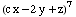after it's expanded.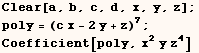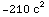We can get the same information from CoefficientList which returns a list of coefficients.  In the cell below Part[coeff, 3,2,5] returns the coefficient of x^(3-1)y^(2-1)z^(5-1).  In general if we say   lst=CoefficientList[poly,{x1,x2,x3,...}]  then  Part[lst,  n1, n2, ,n3, ...] will be the coefficient of x1^(n1-1) x2^(n2-1) x3^(n3-1).The next line gives the coefficient of x^5.  As expected there is more than one term of poly with x^5 as a factor.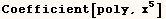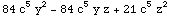We can get the same result as the previous example if we use the next line.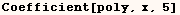One can't get the result above directly from CoefficientList.  Instead pieces of the above result are included in the result of CoefficientList[poly,{x,y,z}].  The line below can be used to get pieces of the result above.  Specifically  coeff[] contains all coefficients of x^5 (including those that are zero).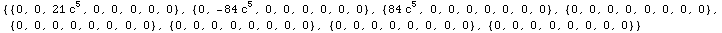Part[coeff, 6, 1, 3] is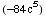the coefficient of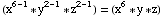in poly. Part[coeff,6,3,1] is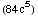the coeficient of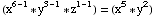in poly.  All other coefficients under Coeff[] are zero which agrees with the result of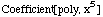.

The function defined in the next cell is a slight modification of a solution Roberto Colistete sent to the MathGroup. This function takes the coefficients from CoefficientList and returns the polynomial with those coefficients.  See the discussions of MapIndexed, and Function for further explanation of some features used here.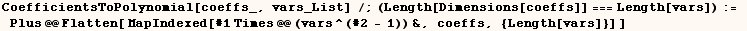The next cell shows another implementation of the same function by Michael Trott of Wolfram Research.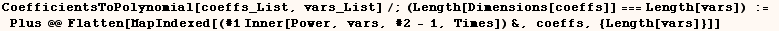A most elegant implementation of the same function is given in the next cell. This is a slight modification of a solution Alan Hayes sent to the MathGroup.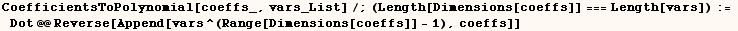Either of the above definitions can be used on the examples below.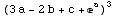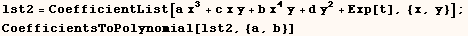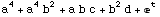Also this hyperlink will take you to a Wolfram Research web site with more information.

Created by Mathematica  (May 16, 2004)

Back to Ted’s Tricks index page